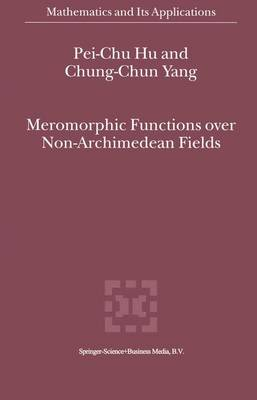•# Meromorphic Functions over Non-Archimedean Fields - Mathematics and Its Applications 522 (Hardback)

(author), (author)
£89.99
Hardback 295 Pages / Published: 30/09/2000
• We can order this

Usually dispatched within 3 weeks

Nevanlinna theory (or value distribution theory) in complex analysis is so beautiful that one would naturally be interested in determining how such a theory would look in the non Archimedean analysis and Diophantine approximations. There are two "main theorems" and defect relations that occupy a central place in N evanlinna theory. They generate a lot of applications in studying uniqueness of meromorphic functions, global solutions of differential equations, dynamics, and so on. In this book, we will introduce non-Archimedean analogues of Nevanlinna theory and its applications. In value distribution theory, the main problem is that given a holomorphic curve f : C -+ M into a projective variety M of dimension n and a family 01 of hypersurfaces on M, under a proper condition of non-degeneracy on f, find the defect relation. If 01 n is a family of hyperplanes on M = r in general position and if the smallest dimension of linear subspaces containing the image f© is k, Cartan conjectured that the bound of defect relation is 2n - k + 1. Generally, if 01 is a family of admissible or normal crossings hypersurfaces, there are respectively Shiffman's conjecture and Griffiths-Lang's conjecture. Here we list the process of this problem: A. Complex analysis: (i) Constant targets: R. Nevanlinna for n = k = 1; H. Cartan  for n = k > 1; E. I. Nochka , , for n > k ~ 1; Shiffman's conjecture partially solved by Hu-Yang [71J; Griffiths-Lang's conjecture (open).

Publisher: Springer
ISBN: 9780792365327
Number of pages: 295
Weight: 1340 g
Dimensions: 235 x 155 x 19 mm
Edition: 2000 ed.